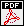## On Kato's local epsilon-isomorphism Conjecture for rank one Iwasawa modules (old title: On the non-commutative Local Main Conjecture for elliptic curves with complex multiplication )

Autors: Otmar Venjakob
Titel:  On Kato's local epsilon-isomorphism Conjecture for rank one Iwasawa modules
Jahr: 2013
Seiten: 42
In: Algebra Number Theory 7 (2013), no. 10, 2369--2416.Abstract: This paper contains a complete proof of Fukaya's and Kato's $\epsilon$-iso\-morphism conjecture in \cite{fukaya-kato} for invertible $\Lambda$-modules (the case of $V = V_0(r)$ where $V_0$ is unramified of dimension $1$). Our results rely heavily on Kato's unpublished proof of (commutative) $\epsilon$-isomorphisms for one dimensional representations of $G_{\qp}$ in \cite{kato-lnmII}, but apart from fixing some sign-ambiguities in (loc.\ cit.) we use the theory of $(\phi,\Gamma)$-modules instead of syntomic cohomology. Also, for the convenience of the reader we give a slight modification or rather reformulation of it in the language of \cite{fukaya-kato} and extend it to the (slightly non-commutative) semi-global setting. Finally we discuss some direct applications concerning the Iwasawa theory of CM elliptic curves, in particular the local Iwasawa Main Conjecture for CM elliptic curves $E$ over the extension of $\qp$ which trivialises the $p$-power division points $E(p)$ of $E$. In this sense the paper is complimentary to the joint work \cite{bou-ven} on noncommutative Main Conjectures for CM elliptic curves.